# 6.1.8 Required Practical: Investigating Reflection & Refraction

### Required Practical 9: Investigating Reflection & Refraction

#### Equipment List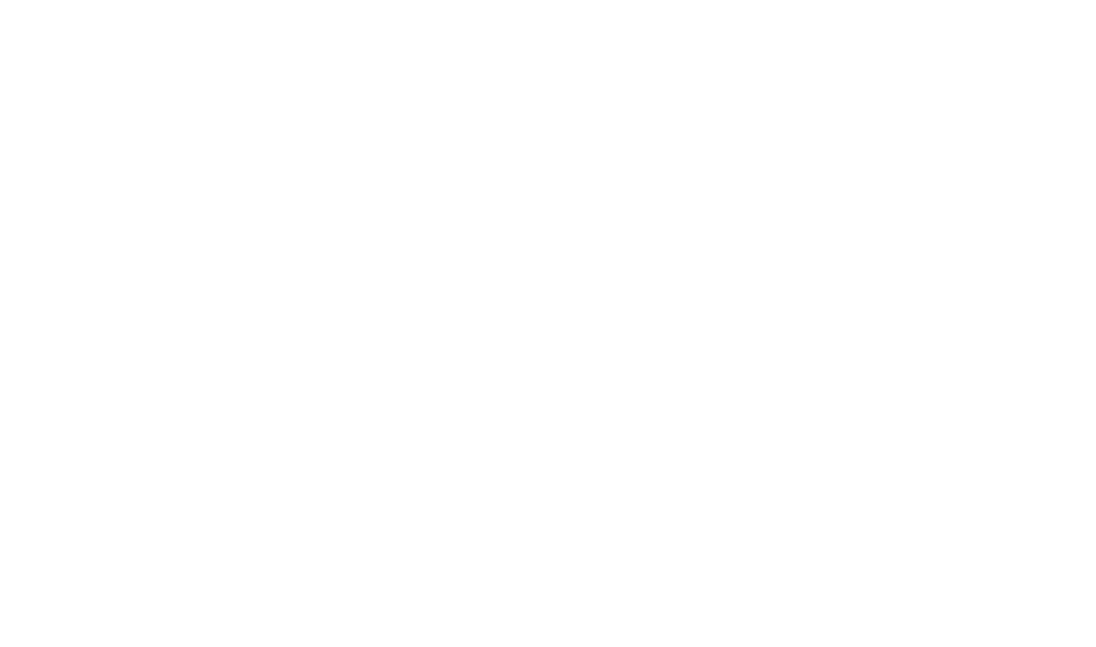• Resolution of measuring equipment:
• Protractor = 1°
• Ruler = 1 mm

#### Aims of the Experiment

• To investigate specular reflection off a smooth surface

Variables

• Independent variable = angle of incidence, i
• Dependent variable = angle of reflection, r
• Control variables:
• Distance of ray box from mirror
• Width of the light beam
• Same frequency / wavelength of the light

#### Method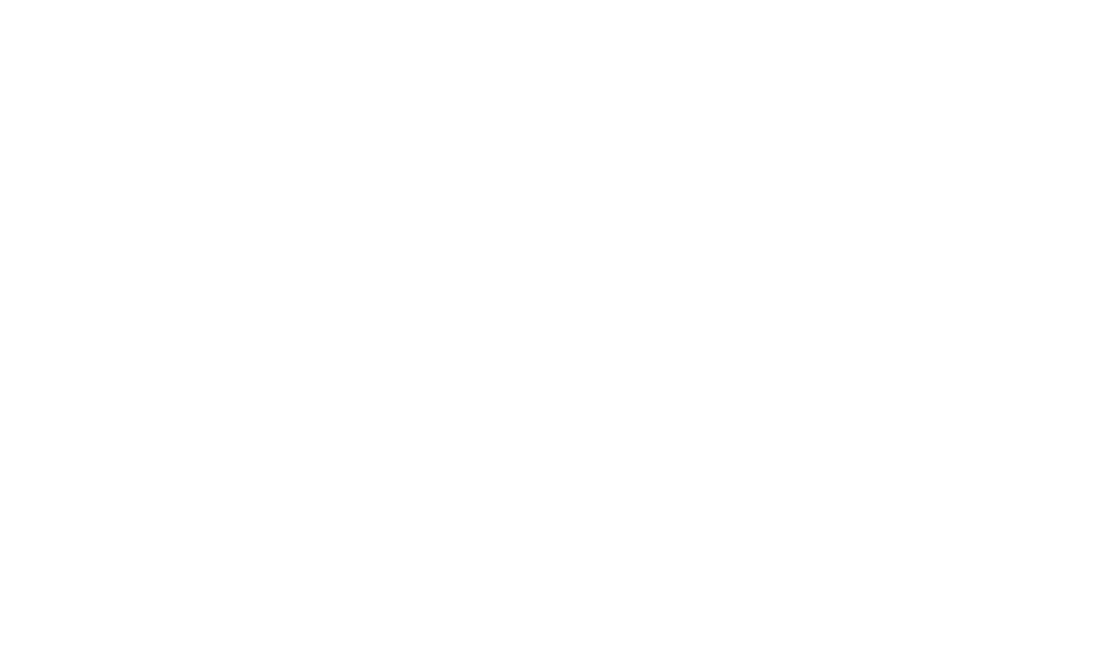Apparatus to investigate reflection

1. Set up the apparatus as shown in the diagram
2. In the middle of the paper use a ruler to mark a straight line of about 10 cm long
3. Use a protractor to draw a 90° line that bisects (cuts in half) the 10 cm line
4. Place the mirror on the first line as shown in the diagram above
5. Switch on the ray box and aim a beam of light at the point where the two drawn lines cross at an angle
6. Use the pencil to mark two positions of the light beam:
• A point just after leaving the ray box
• The point on the reflected beam about 10 cm away from the mirror
7. Remove the ray box and mirror
8. Use a ruler to join the two marked positions to the point where the originally drawn lines crossed
9. Use the protractor to measure the two angles from the 90° line. The angle for the ray towards the mirror is the angle of incidence, and the other the angle of reflection
10. Repeat the experiment three times with the beam of light aimed at different angles
• An example of the data collection table is shown below: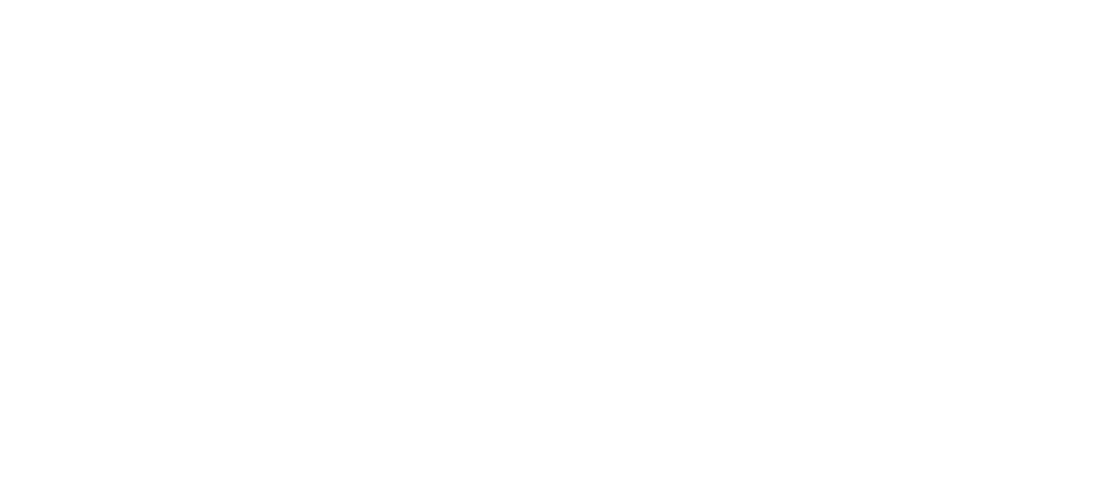#### Analysis of Results

• The law of reflection states:

i = r

• Where:
• i = angle of incidence in degrees (°)
• r = angle of reflection in degrees (°)
• If the experiment was carried out correctly, the angles should be the same, as shown below: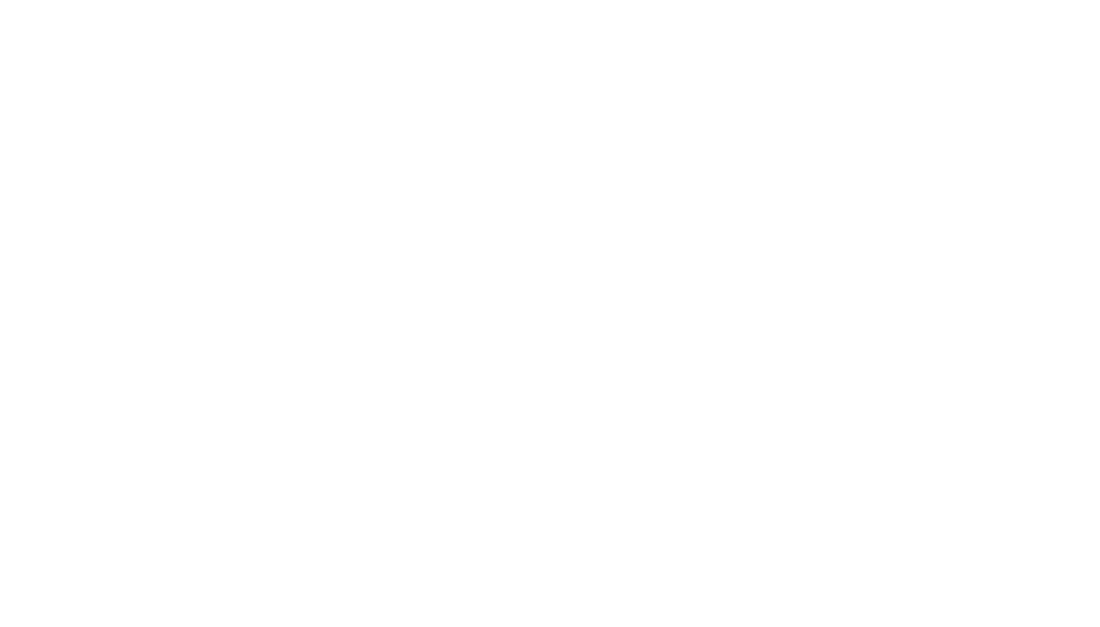Law of reflection

#### Aim of the Experiment

• To investigate the refraction of light by a perspex block

Variables

• Independent variable = angle of incidence, i
• Dependent variable = angle of refraction , r
• Control variables:
• Use of the same perspex block
• Width of the light beam
• Same frequency / wavelength of the light

#### Method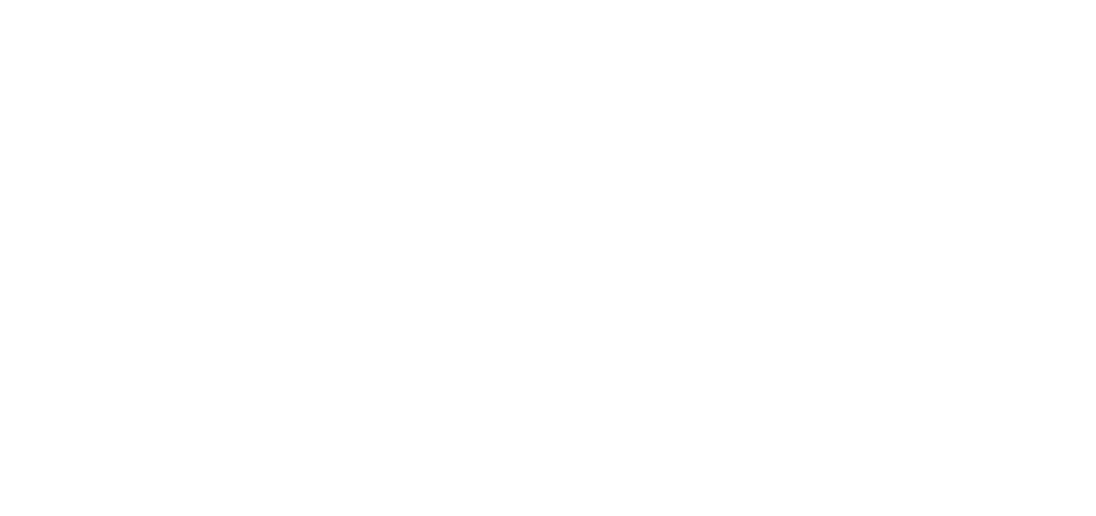Apparatus to investigate refraction

1. Place the glass block on a sheet of paper, and carefully draw around the block using a pencil
2. Switch on the ray box and direct a beam of light at the side face of the block
3. Mark on the paper:
• A point on the ray close to the ray box
• The point where the ray enters the block
• The point where the ray exits the block
• A point on the exit light ray which is a distance of about 5 cm away from the block
4. Draw a dashed line normal (at right angles) to the outline of the block where the points are
5. Remove the block and join the points marked with three straight lines
6. Replace the block within its outline and repeat the above process for a ray striking the block at a different angle
• An example of the data collection table is shown below:#### Analysis of Results

• i and r are always measured from the normal
• For light rays entering perspex block, the light ray refracts towards the central line:

i > r

• For light rays exiting the perspex block, the light ray refracts away from the central line:

i < r

• When the angle of incidence is 90° to the perspex block, the light ray does not refract, it passes straight through the block:

i = r

• If the experiment was carried out correctly, the angles should follow the pattern, as shown below: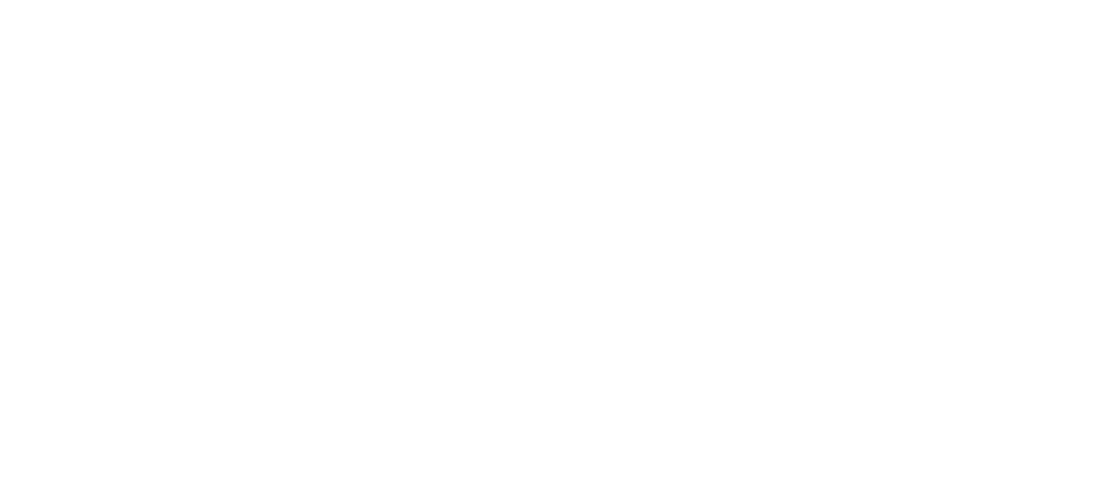How to measure the angle of incidence and angle of refraction

#### Evaluating the Experiment

Systematic Errors:
• An error could occur if the 90° lines are drawn incorrectly
• Use a set square to draw perpendicular lines
• If the mirror is distorted, this could affect the reflection angle, so make sure there are little to no blemishes on it
Random Error
• The points for the incoming and reflected beam may be inaccurately marked
• Use a sharpened pencil and mark in the middle of the beam
• The protractor resolution may make it difficult to read the angles accurately
• Use a protractor with a higher resolution

#### Safety Considerations

• The ray box light could cause burns if touched
• Run burns under cold running water for at least five minute
• Looking directly into the light may damage the eyes
• Avoid looking directly at the light
• Stand behind the ray box during the experiment
• Keep all liquids away from the electrical equipment and paper
• Take care using the mirror
• Damages on the mirror can affect the outcome of the reflection experiment### Author: Katie

Katie has always been passionate about the sciences, and completed a degree in Astrophysics at Sheffield University. She decided that she wanted to inspire other young people, so moved to Bristol to complete a PGCE in Secondary Science. She particularly loves creating fun and absorbing materials to help students achieve their exam potential.
CloseClose